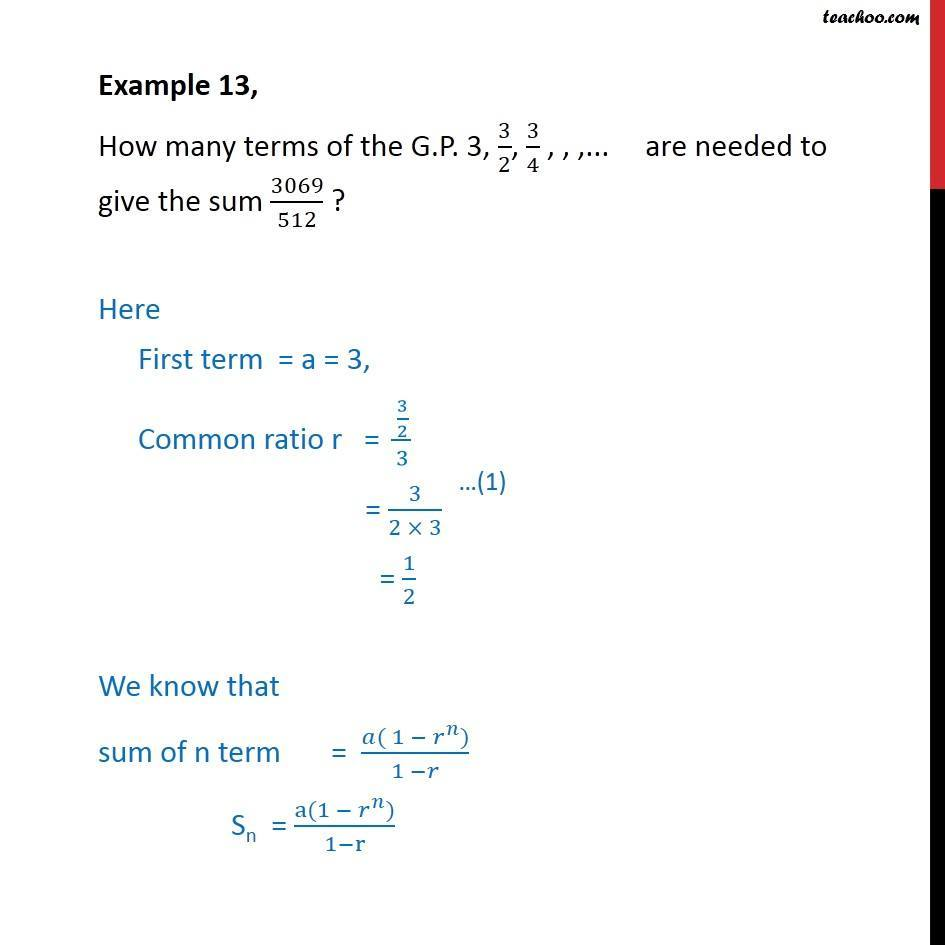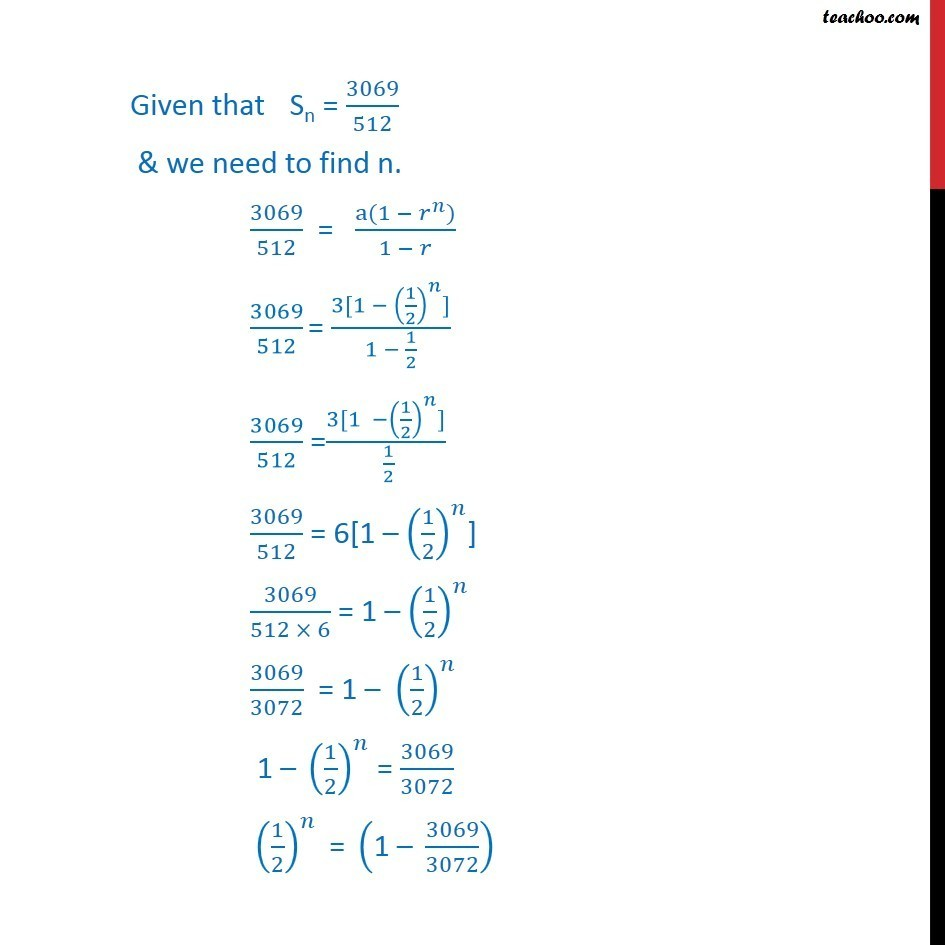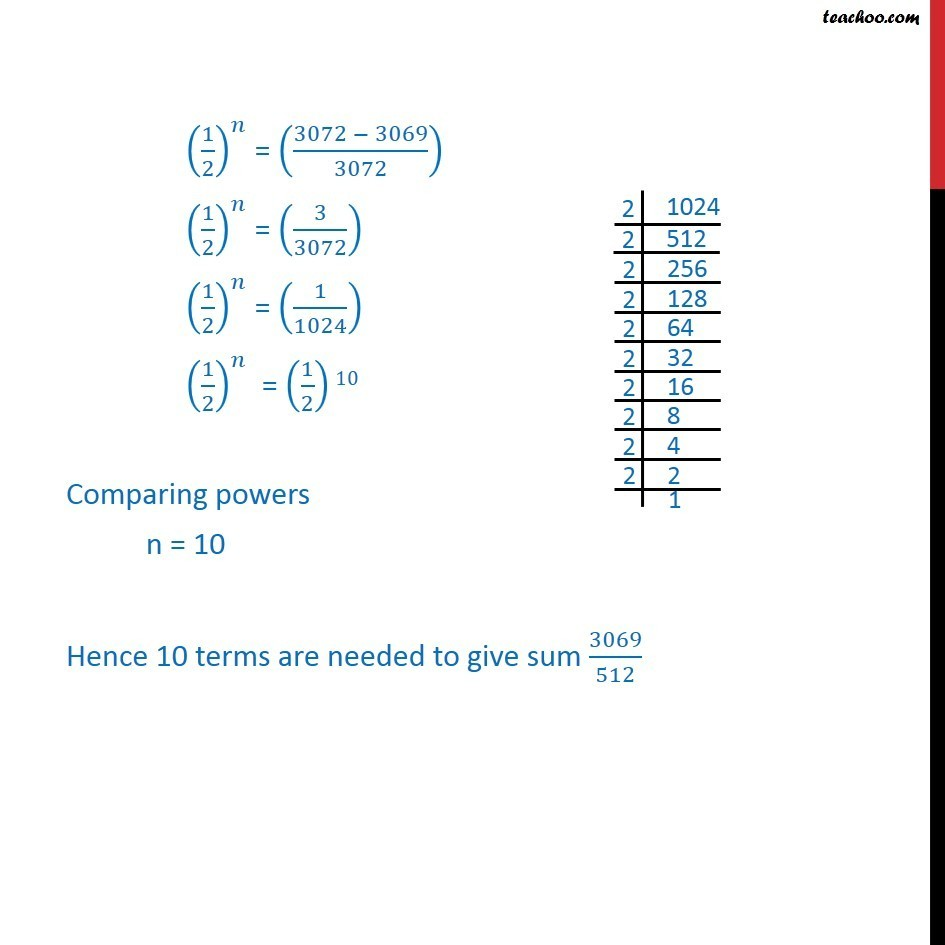Examples

Chapter 8 Class 11 Sequences and Series
Serial order wiseLearn in your speed, with individual attention - Teachoo Maths 1-on-1 Class

### Transcript

Example 8, How many terms of the G.P. 3, 3/2, 3/4 , , ,... are needed to give the sum 3069/512 ? Here First term = a = 3, Common ratio r = (3/2)/3 = 3/(2 3) = 1/2 We know that sum of n term = ( ( 1 ^ ))/(1 ) Sn = (a(1 ^ ))/(1 r) Given that Sn = 3069/512 & we need to find n. 3069/512 = (a(1 ^ ))/(1 ) 3069/512 = (3[1 (1/2)^ ])/(1 1/2) 3069/512 =(3[1 (1/2)^ ])/( 1/2) 3069/512 = 6[1 (1/2)^ ] 3069/(512 6) = 1 (1/2)^ 3069/3072 = 1 (1/2)^ 1 (1/2)^ = 3069/3072 " " (1/2)^ " = " ("1 " 3069/3072) (1/2)^ = ((3072 3069)/3072) (1/2)^ = (3/3072) (1/2)^ = (1/1024) (1/2)^ = (1/2)10 Comparing powers n = 10 Hence 10 terms are needed to give sum 3069/512